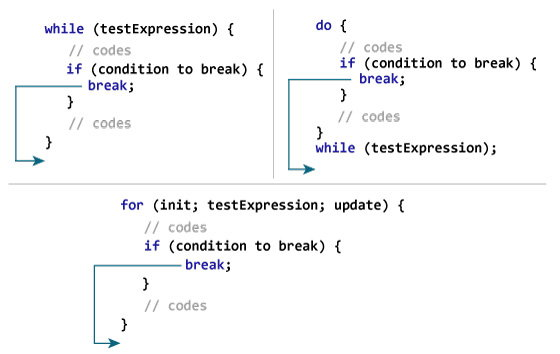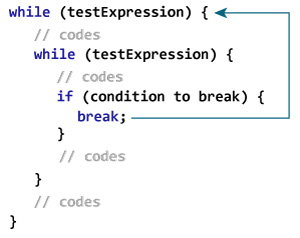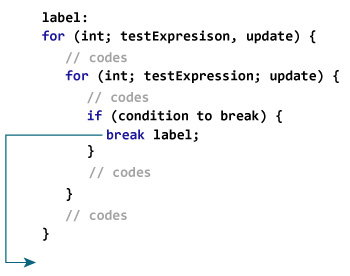# Java break Statement

In this tutorial, you will learn to use break statement to terminate a loop.

Suppose you are working with loops. It is sometimes desirable to skip some statements inside the loop or terminate the loop immediately without checking the test expression.

In such cases, `break` and `continue` statements are used. You will learn about continue statement in the next chapter.

The `break` statement terminates the loop immediately, and the control of the program moves to the next statement following the loop.

It is almost always used with decision making statements (if...else Statement).

The syntax of a break statement is:

`break;`

## How break statement works?### Example 1: Java break statement

``````class Test {
public static void main(String[] args) {

for (int i = 1; i <= 10; ++i) {
if (i == 5) {
break;
}
System.out.println(i);
}
}
}``````

When you run the program, the output will be:

```1
2
3
4```

In the above program, when the value of i becomes 5, expression `i == 5` inside the parenthesis of `if` statement is evaluated to `true`. Then, The `break` statement is executed terminates the for loop.

### Example 2: Java break statement

The program below calculates the sum of numbers entered by the user until user enters a negative number.

To take input from the user, `Scanner` object is used. Visit Java Basic Input to learn more on how to take input from the user.

``````import java.util.Scanner;

class UserInputSum {
public static void main(String[] args) {

Double number, sum = 0.0;
Scanner input = new Scanner(System.in);

while (true) {
System.out.print("Enter a number: ");
number = input.nextDouble();

if (number < 0.0) {
break;
}

sum += number;
}
System.out.println("Sum = " + sum);
}
}``````

When you run the program, you will get similar output like this:

```Enter a number: 3.2
Enter a number: 5
Enter a number: 2.3
Enter a number: 0
Enter a number: -4.5
Sum = 10.5
```

In the above program, the test expression of the `while` loop is always `true`.

Here, the `while` loop runs until user enters a negative number. If user inputs negative number, `break` statement inside the body of `if` statement is executed which terminates the `while` loop.

In case of nested loops, `break` terminates the innermost loop.Here, the break statement terminates the innermost `while` loop, and control jumps to the outer loop.

## Labeled break Statement

The `break` statement we have discussed till now is unlabeled form of break statement, which terminates the innermost `for`, `while`, `do..while` and `switch` statement. There is another form of `break` statement, labeled `break`, that can be used to terminate the outer loop.

### How labeled break statement works?Here, label is an identifier. When `break` statement executes, it terminates the labeled statement, and control of the program jumps to the statement immediately following the labeled statement.

Here's another example:

``````while (testExpression) {
// codes
second:
while (testExpression) {
// codes
while(testExpression) {
// codes
break second;
}
}
// control jumps here
}``````

When `break second;` executes, control jumps to the statement following the labeled statement `second`.

### Example 3: labeled break Statement

``````class LabeledBreak {
public static void main(String[] args) {

first:
for( int i = 1; i < 5; i++) {

second:
for(int j = 1; j < 3; j ++ ) {
System.out.println("i = " + i + "; j = " +j);

if ( i == 2)
break first;
}
}
}
}``````

When you run the program, the output will be:

```i = 1; j = 1
i = 1; j = 2
i = 2; j = 1```

Here's a variation of the above program. The `break` statement in the program below terminates label second, and control jumps to the statement following labeled statement second.

``````class LabeledBreak {
public static void main(String[] args) {

first:
for( int i = 1; i < 5; i++) {

second:
for(int j = 1; j < 3; j ++ ) {

System.out.println("i = " + i + "; j = " +j);

if ( i == 2)
break second;
}
}
}
}``````

When you run the program, the output will be:

```i = 1; j = 1
i = 1; j = 2
i = 2; j = 1
i = 3; j = 1
i = 3; j = 2
i = 4; j = 1
i = 4; j = 2```

The `break` statement is also used to terminate `switch` statement. To learn more, visit Java switch statement.